# Time zero normalization with the Multivariate Gaussian distribution

Timecourses are a powerful experimental design for evaluating the impact of a perturbation. These perturbations are usually chemicals because chemicals, such as a drug, can be introduced quickly and with high temporal precision. Although, with some technologies, such as the estradiol-driven promoters that I used in the induction dynamics expression atlas (IDEA), it is possible to rapidly perturb a single gene further increasing specificity and broadening applicability. By rapidly perturbing individuals, they can be synchronized based on the time when dosing began. We often call this point when dosing begins “time zero” while all subsequent measurements correspond to the time post perturbation. (Since time zero corresponds to a point when a perturbation is applied, but will not yet impact the system, this measurement is usually taken before adding the perturbation.)

One of the benefits of collecting a time zero measurement is it allows us to remove, or account for, effects that are shared among all time points. In many cases this may just amount to analyzing fold-changes of post-perturbation measurement with respect to their time zero observation, rather than the original measurements themselves. This can be useful if there is considerable variation among timecourses irrespective of the perturbation, such as if we were studying humans or mice. Similarly, undesirable variation due to day-to-day variation in instruments, sample stability, or any of the many other factors which could produce batch effects, can sometimes by addressed by measuring each timecourse together and working with fold-changes. In either case, correcting for individual effects using pre-perturbation measurements will increase our power to detect perturbations’ effects.

Aside from correcting for unwanted variation, the kinetics of timecourses are a rich source of information which can be either a blessing or a curse. With temporal information, ephemeral responses can be observed. We can see both which features are changing and when they are changing. And, the ordering of events can point us towards causality. In practice, each of these goals can be difficult, or impossible to achieve, leaving us with a nagging feeling that we’re leaving information on the table. There are many competing options for identifying differences in timecourses, few ways of summarizing dynamics in an intuitive way, and causal inference is often out of reach. In this post, and others to follow, I’ll pick apart a few of these limitations, discussing developments that were applied to the IDEA, but will likely be useful for others thinking about biological timeseries analysis (or other timeseries if you are so inclined!). Here, I evaluate a few established methods for identifying features which vary across time and then introduce an alternative approach based on the Multivariate Gaussian distribution and Mahalanobis distance which increases power and does not require any assumptions about responses’ kinetics.

## Our timecourse experiment

To evaluate methods for detecting temporal dynamics its helpful to use a dataset where there a clear-cut examples of timecourses with and without signal. With such a dataset in hand, we can easily detect signals that we are missing (false negatives), noise that we think is real (false positives) and evaluate overall recall (what fraction of signals are we detecting). We rarely have such positive and negative examples in real timecourses, so instead we can simulate timecourses with and without signal. Going forward I will also use genes as short-hand for whatever features that we might be working with since I’ve primarily worked with these methods in the context of gene expression data.

### Environment Setup

First, I’m going to setup the R environment by loading some bread-and-butter packages and setting the global options for future and ggplot2.

### Simulate timecourses containing signal

First, we can generate the subset of our timecourses which contain signal. These timecourses should follow a broad range of biologically-feasible patterns.

To construct such timecourses, we can use the phenomonological timecourse model of Chechik & Koller which represents timecourses as a pair of sigmoidal responses, called an impulse, We’ll also use a simpler single sigmoidal version of the C & K model.

To simulate data from these models, we can use the simulate_timecourses() function from the impulse R package, available on GitHub.

This function will draw a set of parameters for sigmoidal and impulse from appropriate distributions to define a simulated timecourse. We’ll then add independent normally distributed noise to each observation. (For most genomic data types, measurements are log-normal so we could think of these abundance units as already having been log-transformed).

tc_id time sim_fit abundance signal
2 0 -0.0976223 0.0957832 contains signal
2 5 -0.2018300 -0.5742951 contains signal
2 10 -0.3967604 -0.2166940 contains signal
2 20 -1.1307509 -0.9666770 contains signal
2 30 -1.8594480 -0.9893428 contains signal
2 40 -2.1534230 -1.8150251 contains signal
2 60 -2.2445179 -2.7889178 contains signal
2 90 -2.2489452 -2.5141336 contains signal

### Simulate timecourses which are just noise

Timecourses which are just noise are easy to generate, we can just generate these using independent draws from a normal distribution (with the same standard deviation that we used to add noise to the signals).

With timecourses with and without signals in hand, we can combine the two sets together while tracking their origin.

Additionally since we are interested in time-dependent changes with respect to time zero, we can transform abundances into fold changes which subtract the initial value of a timecourse from every measurement. We’ll work with both the native abundance scale and time-zero normalized fold changes going forward.

### Example timecourses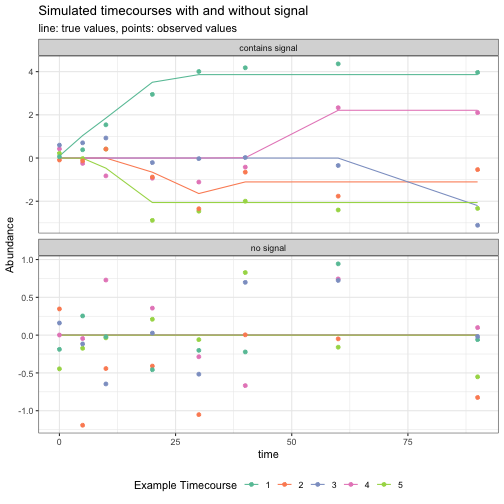## Models to try

At this point, we want to fit a few flavors of time series models to each gene in order to determine how reliably each model can discriminate signal-containing and no-signal timecourses.

To make it easy to iterate over features, I like to using the nest function from tidyr to store all the data for a feature in a single row. Here, expression data will be stored as a list of gene-level tables.

Having nested one-gene per row, we can apply multiple regression models to each gene, and we’ll also do this treating both the fold-change and original expression level as responses to evaluate the effect of time zero normalization. The regression models that we’ll try are:

• linear effect of time on expression. From our sigmoid and impulse generate process, we shouldn’t expect a linear relationship to work great, but it can serve as a nice baseline.
• cubic relationship between time and expression. This fill fit a linear term, a quadratic ($t^2$) and a cubic ($t^3$) term to allow for more complicated dynamics such as genes that go up and then down again. One feature of cubic regression, or other polynomial regression models such as quadratic regression, is that they are zero-centric. What I mean by this is that additional terms in a polyomial regression models, such as moving from a cubic or a quardic model, allows for extra flexibily around zero. This can be helpful, but if changes are occurring at late timepoints, we may need a high degree polynomial to capture this change, and the cost will be a prediction which overfits to the noise in earlier timepoints.
• predicting expression with a spline over timepoints using generalized additive models (GAMs). These models are similar to the cubic models but provide support evenly across time. This will allow them to detect late changes without requiring many degrees of freedom. GAMs are powerful models but they can run into problem when changes occur rapidly especially if we have relatively few timepoints.

With these models our main goal is determine whether each timecourses contains dynamic signal rather than testing for the significance of individual parameters. Approaching the problem this way will also allow us to compare these different approaches even though they fit a different number of parameters. To summarize each model’s prediction of the role of time, ANOVA can be used to determine how much variation is explained by time relative to the noise left over. Because cubic regression and GAMs fit more parameters they must do a better job of explaining the temporal dynamics to justify their extra degrees of freedom.

There are many other approaches that we could try, a few of these are worth mentioning:

• mgcv is an alternative implementation of GAMs to the gam package used below. It is able to decide how flexible of a spline should be fit to each gene using cross-validation. For our synthetic dataset, mgcv actually fails for a number of features owing to the complexity of our dynamics relative to the relatively small number of timepoints.
• If we had replicates of timepoints then we could fit a model which treats each timepoint as a categorical variable. This would allow us detect dynamics without assuming that we expect certain patterns. The downside is that this would require us to collect twice as much data, or perhaps cut back on time points to provide repeated measures of the timepoints that we do have. In general, I think its better to have more unique timepoints represented even if we don’t have repeated measures since this provides more even representation of measuremetns over the time period we care about.
• Since time points are not evenly spaced we could have tried transforming time when fitting the above models. While the timepoints are “exponentially” sampled, taking log(time) would send time zero to -Inf so a better transformation would be using the square root of time as the independent variable.

A couple of notes:

• Since the time zero fold change must be zero by definition, I applied this as a constraint (this is the “+ 0” in the formulas below).
• future was used to parallelize over genes; its settings were setup in the “environment setup” section.

Each model x gene can be summarized by a single p-value. We expect the signal-containing timecourses to have relatively low p-values, while the no-signal timecourses’ pvalues should be uniformly distributed between 0 and 1.

To correct for multiple tests we can use the Storey q-value approach to control the false discovery rate (FDR). To do this we will estimate q-values separately for each model and select a q-value cutoff of 0.1 as a cutoff for significance. At this level we expect that 1/10 of genes with a q-value of less than 0.1 will be from the no-signal group.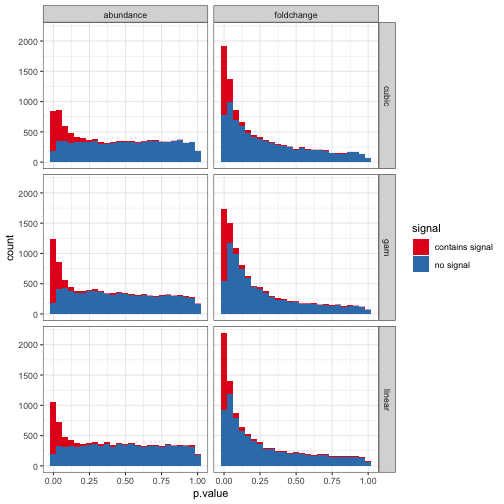Visually, there are a large number of no-signal timecourses with small p-values in the fold-change data. This suggests that something pathological is going on.

We can also summarize models based on the FDR that was actually realized given that we were shooting for 0.1, and based on the total recall of signal-containing timecourses at the cutoff of 0.1

model response false negative false positive true negative true positive fdr recall
cubic abundance 1912 10 7990 88 0.1020408 0.0440
gam abundance 1118 128 7872 882 0.1267327 0.4410
linear abundance 1532 55 7945 468 0.1051625 0.2340
cubic foldchange 280 2928 5072 1720 0.6299484 0.8600
gam foldchange 276 4151 3849 1724 0.7065532 0.8620
linear foldchange 377 3463 4537 1623 0.6808887 0.8115

In this summary we can see that working with abundances does accurately control the FDR, but recall is low for linear and cubic regression and moderate for GAMs. Working with fold changes in contrast fails to control the FDR. While we intended for 1/10 of our discoveries to be null, around 60% actually are! While recall is pretty good, most of the kinetic responses will be garbage.

To figure out what is going wrong, we can plot examples of false positives (model thinks there is signal when there isn’t) and false negatives (model doesn’t think there is signal when there really is).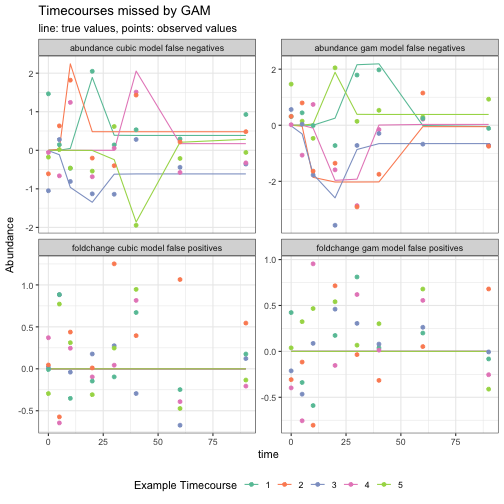From this we can say a few things:

• When working with abundances we need to include an intercept term so the average value of a feature can be separated from its change over time. Doing this however can remove some early responses since the intercept becomes the point of reference rather than the value at time zero.

• Changes which are showing up primarily in one or two timepoints might be missed since the polynomial and gam models used above can’t contort themselves to fit these dynamics. This might be appropriate if these points were just noise but in many cases these are large changes beyond what we would expect as noise in our generative process.

• Working with fold change enforces the value at time zero as the appropriate reference. This makes conceptual sense for a perturbation timecourse since at time zero (and before) the system is in a reference state, and all subsequent timepoints capture the dynamics of interest. However, working directly with fold-changes creates a problem. We are no longer controlling the FDR! In this simulation if we wanted to find discoveries at a 10% FDR than we would in fact be realizing a 60% FDR. This is a big problem, especially since outside of working in a simulation, we don’t know which timecourses contain real signal and which are spurious (if that was the case then why would we do the experiment…), so we would would think there were strong signals in our dataset when it may in fact entirely be noise.

If we wanted to get around these issues, then we could still probably make a regression model work, but it would require adding more samples and cost to the analysis. The two main paths we could take are:

• replicates of each timecourse - if we had multiple biological replicates at each timepoint, than rather than treating time as a numerical variable, we could treat it as a categorical variable. In this case we could fit an ANOVA model which would assess whether the variation between timepoints is greater than the variation within timepoints. This would be a powerful way of detecting differences across time which is agnostic to types of changes occurring.

• denser sampling - if we had more measurements near timepoints where rapid dynamics were occurring then it would be easier to distinguish smooth rapid responses from single outlier observations. This would still require us to fit a model which can appropriately capture such dynamics, but with more observations we could either fit a more flexible model (with more degrees of freedom) or use the same simple models but with more power to detect significant changes.

In most cases, I think adopting one of these options is probably the smart way to go, however there are reasons why collecting more samples in a given experiment is not feasible such as if MANY similar experiments are being performed, like in IDEA, or if there are constraints on how frequently samples can be collected.

In such cases, I think we can find a path forward by stepping away from regression and thinking about likelihood-based methods which capture the nature of fold changes.

## Timecourse fold change likelihood

Using likelihood methods, we start with a statistical model for how our observations were generated. We can then sample or optimize the parameters of this model in order to find the most likely value (i.e., the frequentist MLE) or generate a distribution of parameters incorporating both likelihood and parameter plausibility (i.e., the Bayesian approach).

Before we posit an appropriate likelihood for fold change, lets figure out why the regression approaches using fold change were so anticonservative. The big problem here was that many timecourses which were just noise looked like they actually contained signal. So, lets work with just the “no signal” timecourses.

To do this, we can look at how the value at time zero influences fold-change estimates of later timepoints.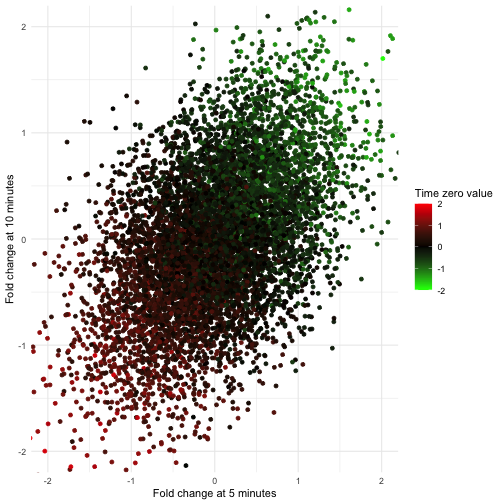From this plot, a large positive value at time zero (due to noise) results in later timepoints appearing as consistent negative fold changes. Conversely, if the time zero value is negative then later timepoints appear consistently positive.

While we simulated abundances as independent Normal draws which would possess a spherical covariance structure, normalizing to time zero has induced a correlation between observations and this dependence will need to be accounted for in order to have a useful null hypothesis.

Based on the value of time zero, which all subsequent time points are normalized with respect to, these later timepoints are biased such that they are all higher or lower than otherwise expected. In order to test for timecourse-level signal based on the aggregate signal of all observations, we need to account for the dependence of these observations. Luckily, the form of this dependence is quite straight-forward.

We can see this dependence using the sample covariance matrix of our null timecourses.

5 10 20 30 40 60 90
5 0.5141863 0.2514794 0.2583391 0.2533660 0.2557825 0.2539230 0.2559496
10 0.2514794 0.5076230 0.2605876 0.2536349 0.2504740 0.2512202 0.2540698
20 0.2583391 0.2605876 0.5131240 0.2505837 0.2562055 0.2559705 0.2547911
30 0.2533660 0.2536349 0.2505837 0.4973020 0.2534935 0.2517957 0.2526950
40 0.2557825 0.2504740 0.2562055 0.2534935 0.4982398 0.2498487 0.2531005
60 0.2539230 0.2512202 0.2559705 0.2517957 0.2498487 0.4986771 0.2508796
90 0.2559496 0.2540698 0.2547911 0.2526950 0.2531005 0.2508796 0.5066272

Observations variances are approximately $2\text{Var}(x_t)$ (2 * 0.5) because:

$\mathcal{N}(\mu_{A}, \sigma^{2}_{A}) - \mathcal{N}(\mu_{B}, \sigma^{2}_{B}) = \mathcal{N}(\mu_{A} - \mu_{B}, \sigma^{2}_{A} + \sigma^{2}_{B})$

Observation covariances are $\text{Var}(\log_2x_t)$ because the shared normalization to time zero adds the variance of time zero as a covariance to to the later time points.

Normalization of Normal (or log-Normal) observations to a common reference produces a Multivariate Gaussian distribution.

$\mathbf{f}_{i} \sim \mathcal{MN}\left(\mu = \mathbf{0}, \Sigma = \begin{bmatrix} 2\sigma_{\epsilon}^2 & \sigma_{\epsilon}^2 & \dots & \sigma_{\epsilon}^2 \\ \sigma_{\epsilon}^2 & 2\sigma_{\epsilon}^2 & \dots & \sigma_{\epsilon}^2 \\ \vdots & \vdots & \ddots & \vdots \\ \sigma_{\epsilon}^2 & \sigma_{\epsilon}^2 & \dots & 2\sigma_{\epsilon}^2 \end{bmatrix}\right)$

If we expect null fold-change measurements to follow this distribution, then we can sample from a multivariate normal distribution to explore whether this works as a fold-change generative process. We can then assess whether these draws are likely to have come from this distribution as a null hypothesis. For this purpose, we’ll use Mahalanobis distance, which is a multivariate generalization of the Wald test that assesses how many standard deviations an observation is from the mean of the distribution. This requires an estimate of the covariance matrix, an assumption that will be discussed below. We expect these statistics to be $\chi^{2}$ distributed with degrees of freedom equal to the number of timepoints that we have.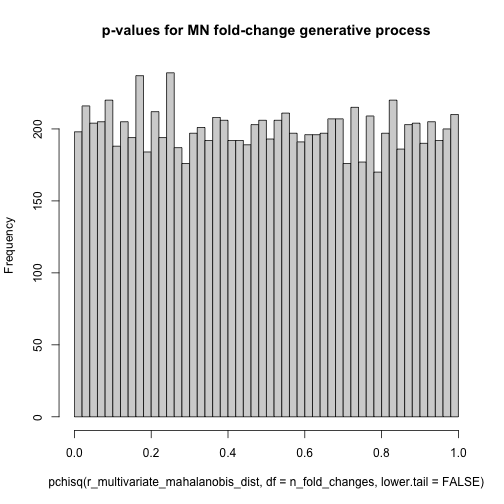Having taken draws from the Multivariate Gaussian distribution and then used the Mahalanobis distance to calculate p-values, the $\text{Unif}(0,1)$ distribution of these p-values confirms that the Mahalanobis distance is appropriate.

We can now test whether fold-changes are really Multivariate Gaussian distributed by inspecting the distribution of Mahalanobis distance p-values from the no-signal timecourses.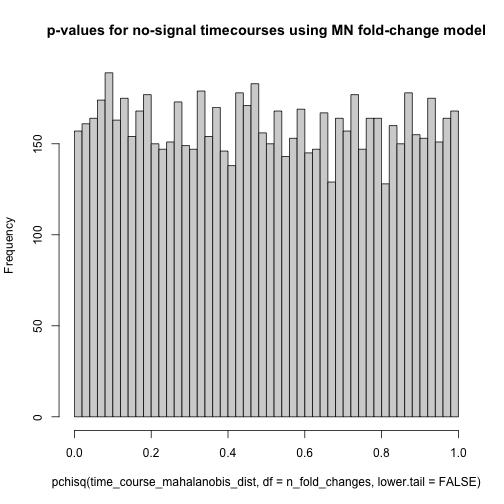The p-values for the no-signal fold change timecourses are indeed $\text{Unif}(0,1)$ distributed as we hoped.

Now, we can calculate the Mahalanobis distances and their corresponding p-values for the signal-containing timecourses as well. Signal in these timecourses will both increase the overall variance in expression for a feature, and deviations of nearby timepoints may be similar. These factors will make it harder for the Multivariate Gaussian noise model to explain the signal-containing expression vector, resulting in a high Mahalanobis distance and a small p-value.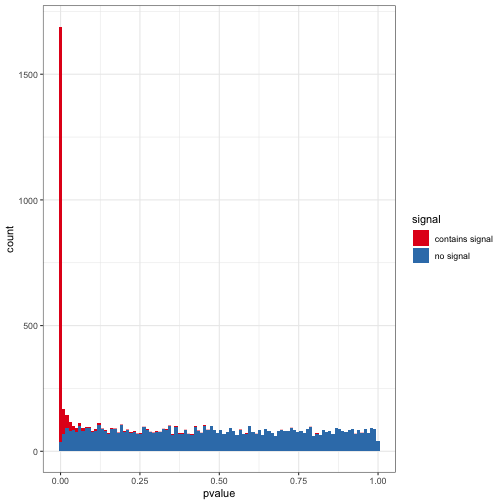Based on the p-value distributions, most of the signal-containing timecourses have small p-values suggesting increased recall. We can verify this as before by summarizing results based on the realized FDR and the overall recall of signal-containing timecourses at this FDR cutoff.

false negative false positive true negative true positive fdr recall
201 194 7806 1799 0.0973407 0.8995

The Multivariate Gaussian test did a great job of appropriately identifying signal-containing timecourses. The high power of this test arises from using estimates of noise level of observations, and our resulting ability to step away from assumptions regrading the types of time-dependent signals we expect.

The test uses not just a pattern of expression but also information about the magnitude of noise associated with each observation. This information is available in many contexts in genomics. A couple examples where observation-level estimates of noise are available are (1) via the mean-variance relationships of RNAseq data or (2) via consistency of peptides in proteomics data. Having these estimates can identify large fold-changes which are unlikely to occur by chance, even if all post time zero timepoints are similar. Similarly, complex rapid dynamics will look like a poorly fit regression model with high residual error. But, if we know the magnitude of residual error that we expect, then signals can be identified by just looking for an excess of variation in the timecourse (accounting for the bias introduced by time zero normalization). Using noise estimates may seem like cheating since this information was not directly used by the other tests. If we were to use an estimate of the noise level it would be to carry-out a weighted least squared regression. But, since all observations have the same level of noise added, here, weighted regressions would be equivalent to the un-weighted regressions used above.

Using Mahalanobis distance, we can look for any departures from the null noise model to define signal. This lets us step away from models which look for particular types of signals, such as the linear, cubic, or smooth relationships sought in the regression models we applied. Some of these models can be quite flexible, but when the underlying data does not follow these relationships these models will often fail. In this case, we simulated signals as biologically feasible sigmoidal or impulse responses, so none of the regression models applied could capture every instance of a simulated signals containing timecourse. In this case, if we were to use a regression model then we would be best off fitting a non-linear least squares model following the sigmoidal or impulse form. Doing this is actually non-trivial if we want to avoid pathological fits, but these issues have been addressed in the impulse R package. I’ll discuss this problem in a future post.